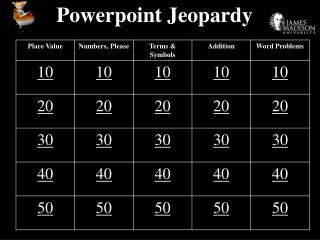DownloadDownload PresentationPowerpoint Jeopardy

# Powerpoint Jeopardy

Download Presentation## Powerpoint Jeopardy

- - - - - - - - - - - - - - - - - - - - - - - - - - - E N D - - - - - - - - - - - - - - - - - - - - - - - - - - -
##### Presentation Transcript

1. Powerpoint Jeopardy

2. How many hundreds are in the number 487?

3. What is the value of the 1 in 601, 346?

4. What is the largest number you can make with the digits 8, 0, 9, 1, and 6?

5. What number is one more than 999,999?

6. How would you write two thousand, seven hundred forty-five?

7. How many minutes are in one hour?

8. How many zeros are in one million?

9. How many days are in four weeks?

10. How many degrees are in a right angle?

11. How many years in a century?

12. What is the figure with three sides and three angles called?

13. What does the symbol < mean?

15. What operation does the symbol x mean?

16. What is the name of a five-sided figure?

17. 29 + 77

18. 93 + 55

19. 65 14 + 8

20. 406 + 631

21. 4,708 + 9,384

22. The pet store has three parrots, 5 canaries and 6 parakeets. How many birds are there in all?

23. There are 24 blue marbles and 17 green marbles. How many more blue marbles are there than green marbles?

24. Kyle has 2 quarters, 2 dimes, 2 nickels and 2 pennies. What is the total amount of money Kyle has?

25. There are 18 teams in a soccer league. Each team has 8 players. How many players are there all together?

26. There are 40 legs in Mrs. Brantley’s class. Each student has 2 legs. How many students are in Mrs. Brantley’s class?